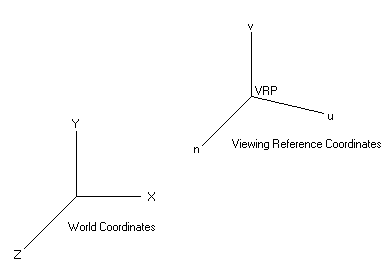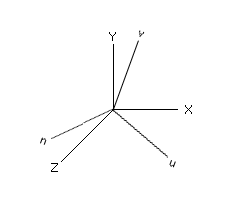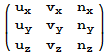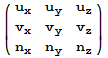# 3D Computer Graphics, Rotations, and Lazy ProfessorsA standard text on computer graphics is "Introduction to Computer Graphics" by Foley, Van Dam, Feiner, Hughes, and Phillips. Even though it was published in 1997, it covers many core techniques that are still useful for writing graphics programs today. No book is perfect, yet Foley's treatment of rotation matrices leaves something to be desired. Depending on one's interests, this may not be a major concern. But one can easily find lecture notes (on the internet) for graduate level computer graphics courses that repeat Foley's treatment of rotations almost verbatim. And that is just lazy -- at the graduate level one expects, well, a graduate level presentation... so I am presenting a more mathematically correct treatment of rotation matrices as they apply to the computer graphics pipeline.
World Coordinates and the Viewing-Reference Coordinates
The situation is we have two coordinate systems, shown below. (To fix ideas, we are thinking of the coordinates as elements of R3, not R4 as a projective space. Then standard linear algebra applies.)The world coordinate system is how our objects are originally specified in 3 dimensions. The viewing-reference coordinate system's claim to fame is that it contains the uv-plane, which is the viewing plane (or projection plane). The viewing plane is of course 2 dimensional, and ultimately part of it will be used to represent the 2D computer screen on which the user will see the original 3D world rendered. The viewing plane is defined by specifying a point on the plane, the view reference point (VRP), and a direction which will be taken as normal to the viewing plane, which in the diagram is indicated by the direction along the n-axis. The v-axis specifys the up direction, and is orthogonal to n. Once the n and v directions are known, then the u-axis is defined so that u,v, and n form a right-handed coordinate system.
So, we will be working in the viewing reference coordinate system and thus we have to perform the following operations:
• Step 1: Translate the viewing-reference axes to the world coordinate axes
so they share the same origin.
• Step 2: Rotate the viewing-reference axes so that the coordinate systems axes are parallel.
The translation indicated in step 1 is routine, and the result is shown below:Step 2 is what concerns us and is where Foley can be improved on. Our task is to figure out how to rotate the the view coordinate system into the world coordinate system.
To define a linear transformation (and a rotation is a linear transformation) it is sufficient to specify its action on any basis. So,

Let {i,j,k} be the standard ordered basis of R3.
Let {u,v,n} be unit vectors along the u,v, and n axes. They also are a basis of R3.
Then the rotation T: R3 → R3 is defined by

u |→ i,
v |→ j,
n |→ k

The first thing to note is we are done. That's it. T is completely specified. Given any vector α ∈ R3, we can expand it as α = a u + b v + c n ,  and the rotation acting on α is then

T(α) = T(a u + b v + c n)
= aT(u) + bT(v) + cT(n)
= ai + bj + ck

The matrix A of a linear transformaion T:Rn → Rn relative to an ordered basis β = {β1,β2,...,βn} is defined by

T(βj) = Σ Aij βi       (j=1,2,..,n)

Notice that the matrix of the transformation is defined by specifying the action of the transformation on a basis, and as I have already indicated, we have been given that in the problem statement.  Incidentally this is the standard method, and it is not the method Foley uses. Foley's method will work to determine the matrix with respect to an orthonormal basis, but it does not work for an arbitrary basis. If you can guarantee your students are only going to work with rotations in R3, and only with orthonormal bases, and not arbitrary linear transformations and bases, then perhaps Foley might be OK, but that is quite an assumption. Better to learn how to do it in the general case.
So, let's make the construction of the matrix A explicit:

T-1(i)  = ux  + uyj + uzk
T-1(j)  = vx  + vyj + vzk
T-1(k) = vx  + vyj + vzk

and so the matrix of T-1 (not T, but the inverse of T) relative to the standard basis is given by:Again, that is the matrix of T-1, not T. But we want the matrix of T. To get that, we finally use the fact that rotations are othogonal transformations, which, by definition means that TTt= I, where Tt is the transpose of T.
Thus,

Tt = T-1

and so,

T=  (T-1)t

Finally, taking the transpose of the matrix above for T-1 , we get the desired matrix for T:and that is the result Foley gets for the rotation matrix relative to the standard basis of R3.